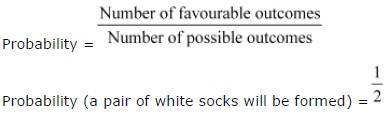# Solutions of Chapter Data handling Exercise-3.1

Question 1: Find the range of heights of any ten students of your class. Answer:

Let the heights (in cm) of 10 students of our class be 125, 127, 132, 133, 134, 136,138, 141, 144, 146

Highest value among these observations = 146

Lowest value among these observations = 125

Range = Highest value − Lowest value

= (146 − 125) cm

= 21 cm

Question 2: Organise the following marks in a class assessment, in a tabular form.

(i) Which number is the highest?

(ii) Which number is the lowest?

(iii) What is the range of the data?

(iv) Find the arithmetic mean.

According to the question,

At first,

We will draw the frequency table of the given data.

Hence,

(i) Highest number = 9

(ii) Lowest number = 1

(iii) Range = (9 − 1) = 8

(iv) Sum of all the observations = 4 + 6 + 7 + 5 + 3 + 5 + 4 + 5 + 2 + 6 + 2

+ 5 + 1 + 9 + 6 + 5 + 8 + 4 + 6 + 7

= 100

Total number of observations = 20

Question 3:

Find the mean of the first five whole numbers.

First five whole numbers are 0, 1, 2, 3, and 4.

Therefore, the mean of first five whole numbers is 2.

Question 4:

A cricketer scores the following runs in eight innings:

58, 76, 40, 35, 46, 45, 0, 100

Find the mean score.

Runs scored by the cricketer are 58, 76, 40, 35, 46, 45, 0, and 100.

Therefore, mean score is 50. Question 5:

Following table shows the points of each player scored in four games:

(i) Find the mean to determine A’s average number of points scored per game.

(ii) To find the mean number of points per game for C, would you divide the total points by 3 or by 4? Why?

(iii) B played in all the four games. How would you find the mean?

(iv) Who is the best performer?

(ii) To find the mean number of points per game for C, we will divide the total points by 3 because C played 3 games.

(iv) The best performer will have the greatest average among all. Now we can observe that the average of A is 12.5 which is more than that of B and C. Therefore, A is the best performer among these three.

Question 6:

The marks (out of 100) obtained by a group of students in a science test are 85, 76, 90, 85, 39, 48, 56, 95, 81 and 75. Find the:

(i) Highest and the lowest marks obtained by the students.

(ii) Range of the marks obtained.

(iii) Mean marks obtained by the group.

The marks obtained by the group of students in a science test can be arranged in an ascending order as follows.

39, 48, 56, 75, 76, 81, 85, 85, 90, 95

(i) Highest marks = 95

Lowest marks = 39

(ii) Range = 95 − 39

= 56

## Question 1:

The scores in mathematics test (out of 25) of 15 students is as follows:

19, 25, 23, 20, 9, 20, 15, 10, 5, 16, 25, 20, 24, 12, 20

Find the mode and median of this data. Are they same?

Scores of 15 students in mathematics test are

19, 25, 23, 20, 9, 20, 15, 10, 5, 16, 25, 20, 24, 12, 20

Arranging these scores in an ascending order,

5, 9, 10, 12, 15, 16, 19, 20, 20, 20, 20, 23, 24, 25, 25

Mode of a given data is that value of observation which occurs for the most number of times. Median of a given data is the middle observation when the data is arranged in an ascending or descending order.

As there are 15 terms in the given data, therefore, the median of this data will be the 8th observation.

Hence, median = 20

Also, it can be observed that 20 occurs 4 times (i.e., maximum number of times).

Therefore, mode of this data = 20

Yes, both are same.

Question 2:

The run scored in a cricket match by 11 players is as follows:

6, 15, 120, 50, 100, 80, 10, 15, 8, 10, 15

Find the mean, mode and median of this data. Are the three same?

The runs scored by 11 players are

6, 15, 120, 50, 100, 80, 10, 15, 8, 10, 15

Arranging these scores in an ascending order,

6, 8, 10, 10, 15, 15, 15, 50, 80, 100, 120

Mode of a given data is that value of observation which occurs for the most number of times and the median of the given data is the middle observation when the data is arranged in an ascending or descending order.

As there are 11 terms in the given data, therefore, the median of this data will be the 6th observation.

Median = 15

Also, it can be observed that 15 occurs 3 times (i.e., maximum number of times).

Therefore, mode of this data = 15

No, these three are not same.

Question 3:

The weights (in kg.) of 15 students of a class are:

38, 42, 35, 37, 45, 50, 32, 43, 43, 40, 36, 38, 43, 38, 47

(i) Find the mode and median of this data.

(ii) Is there more than one mode?

The weights of 15 students are

38, 42, 35, 37, 45, 50, 32, 43, 43, 40, 36, 38, 43, 38, 47

Arranging these weights in ascending order,

32, 35, 36, 37, 38, 38, 38, 40, 42, 43, 43, 43, 45, 47, 50

(i)Mode of a given data is that value of observation which occurs for the most number of times and the median of the given data is the middle observation when the data is arranged in an ascending or descending order.

As there are 15 terms in the given data, therefore, the median of this data will be the 8th observation.

Hence, median = 40

Also, it can be observed that 38 and 43 both occur 3 times (i.e., maximum number of times).

Therefore, mode of this data = 38 and 43

(ii)Yes, there are 2 modes for the given data.

Question 4:

Find the mode and median of the data: 13, 16, 12, 14, 19, 12, 14, 13, 14

The given data is

13, 16, 12, 14, 19, 12, 14, 13, 14

Arranging the given data in an ascending order,

12, 12, 13, 13, 14, 14, 14, 16, 19

Mode of a given data is that value of observation which occurs for the most number of times and the median of the given data is the middle observation when the data is arranged in an ascending or descending order.

As there are 9 terms in the given data, therefore, the median of this data will be the 5th observation.

Hence, median = 14

Also, it can be observed that 14 occurs 3 times (i.e., maximum number of times).

Therefore, mode of this data = 14

Question 5:

Tell whether the statement is true or false:

(i) The mode is always one of the numbers in a data.

(ii) The mean is one of the numbers in a data.

(iii) The median is always one of the numbers in a data.

(iv) The data 6, 4, 3, 8, 9, 12, 13, 9 has mean 9.

(i) True

The given statement is true.

Since,

We know that,

Mode is termed as that value of observation which occurs for the maximum number of times.

Hence,

Mode of a given data is that value of observation which occurs for the most number of times. Therefore, it is one of the observations given in the data.

(ii) False

Mean may or may not be one of the numbers in the data.

(iii) True

The given statement is true.

Since,

We know that,

Median of any data is known as the middle observation when that data is arranged in ascending or descending order.

Hence,

Median is always one of the numbers in a data

Therefore,

The median of the given data is the middle observation when the data is arranged in an ascending or descending order.

(iv) False

The given data is 6, 4, 3, 8, 9, 12, 13, 9

## Question 1:

Use the bar graph (see the given figure) to answer the following questions.

(a) Which is the most popular pet?

(b) How many students have dog as a pet?

(a) Here,

According to the question,

We have to find the most popular pet among them.

Now,

Here,

The bar graph represents the pets owned by the students of class seven.

And,

We can see that,

The bar of the cat is the tallest

Hence,

Cat is the most popular pet.

Since the bar representing cats is the tallest, cat is the most popular pet.

(b) Here,

According to the question,

We have to find the number of students who have dog as a pet.

Now,

Here,

We know that,

The bar graph represents the pets owned by the students of class seven.

And,

We can see that,

The bar of the dog is till 8

Hence,

8 students have dog as their pet.

The number of students having dog as a pet are 8.

Question 2:

Read the bar graph (see the given figure) which shows the number of books sold by a bookstore during five consecutive years and answer the questions that follow:

(i) About how many books were sold in 1989? 1990? 1992?

(ii) In which year were about 475 books sold? About 225 books sold?

(iii) In which years were fewer than 250 books sold?

(iv) Can you explain how you would estimate the number of books sold in 1989?

(i) Here,

We can say that,

While observing the graph,

The total books sold in the year 1989, 1990 and 1992 are as follows:

Number of books sold in 1989 = 175

And,

Number of books sold in 1992 = 225

And,

Number of books sold in 1990 = 475

In 1989, 175 books were sold. In 1990, 475 books were sold. In 1992, 225 books were sold.

(ii) Here,

We have to find,

The year in which the following number of books were sold:

475

And,

225

Hence,

While observing the graph,

We can say that,

475 books were sold in the year 1990

And,

225 books were sold in the year 1992

From the graph, it can be concluded that 475 books were sold in the year 1990 and 225 books were sold in the year 1992.

(iii)Here,

We have to find,

The years in which the books sold were less than 250 books

Hence,

While observing the graph,

We can conclude that,

The years in which the total number of books sold was less than 250 are as follows:

The year 1989 And The year 1992

From the graph, it can be concluded that in the years 1989 and 1992, the number of books sold were less than 250.

(iv) From the graph, it can be concluded that the number of books sold in the year 1989 is about 1 and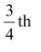part of 1 cm.

We know that the scale is taken as 1 cm = 100 books.

Therefore, about 175 books were sold in the year 1989.

Question 3:

Number of children in six different classes are given below. Represent the data on a bar graph.

(a) How would you choose a scale?

(i) Which class has the maximum number of children? And the minimum?

(ii) Find the ratio of students of class sixth to the students of class eight.

(a) We will choose a scale as 1 unit = 10 children because we can represent a more clear difference between the number of students of class 7th and that of class 9th by this scale.

(b) (i) Since the bar representing the number of children for class fifth is the tallest, there are maximum number of children in class fifth. Similarly, since the bar representing the number of children for class tenth is the smallest, there are minimum number of children in class tenth.

(ii) The number of students in class sixth is 120 and the number of students in class eighth is 100.

Therefore, the ratio between the number of students of class sixth and the number of

Question 4:

The performance of students in 1st Term and 2nd Term is given. Draw a double bar graph choosing appropriate scale and answer the following:

(i) In which subject, has the child improved his performance the most?

(ii) In which subject is the improvement the least?

(iii) Has the performance gone down in any subject?

A double bar graph for the given data is as follows.

(i) Here,

We can see that,

Math had a maximum increase in marks.

Hence,

It can be concluded that,

The child had improved his performance the most in Maths.

There was a maximum increase in the marks obtained in Maths. Therefore, the child has improved his performance the most in Maths.

(ii) Here,

We can see that,

S. science had the least increase in marks.

Hence,

It can be concluded that,

The child had improved his performance the least in S. science.

From the graph, it can be concluded that the improvement was the least in S. Science.

(iii) Here,

We can observe that,

From the given graph,

Hindi has a decrement in marks in the second term

Hence,

It can be concluded that,

The child’s performance has gone down in Hindi.

From the graph, it can be observed that the performance in Hindi has gone down.

Question 5:

Consider this data collected from a survey of a colony.

(i) Draw a double bar graph choosing an appropriate scale. What do you infer from the bar graph?

(ii) Which sport is most popular?

(iii) Which is more preferred, watching or participating in sports?

(i)A double bar graph for the given data is as follows.

(i) Draw a double bar graph choosing an appropriate scale. What do you infer from the bar graph?

(ii) Which sport is most popular?

(iii) Which is more preferred, watching or participating in sports?

(i)A double bar graph for the given data is as follows.

The double bar graph represents the number of people who like watching and participating in different sports. It can be observed that most of the people like watching and participating in cricket while the least number of people like watching and participating in athletics.

(ii) From the bar graph, it can be observed that the bar representing the number of people who like watching and participating in cricket is the tallest among all the bars. Hence, cricket is the most popular sport.

(iii) The bars representing watching sport are longer than the bars representing participating in sport. Hence, watching different types of sports is more preferred than participating in the sports.

Question 6:

Take the data giving the minimum and the maximum temperature of various cities given in the following table:

Temperatures of the cities as on 20.6.2006

Plot a double bar graph using the data and answer the following:

(i) Which city has the largest difference in the minimum and maximum temperature on the given date?

(ii) Which is the hottest city and which is the coldest city?

(iii) Name two cities where maximum temperature of one was less than the minimum temperature of the other.

(iv) Name the city which has the least difference between its minimum and the maximum temperature.

A double bar graph for the given data is constructed as follows.

(i) From the graph, it can be concluded that Jammu has the largest difference in its minimum and maximum temperatures on 20.6.2006.

(ii) From the graph, it can be concluded that Jammu is the hottest city and Bangalore is the coldest city.

(iii) Bangalore and Jaipur, Bangalore and Ahmedabad

For Bangalore, the maximum temperature was 28ºC, while minimum temperature of both cities, Ahmedabad and Jaipur, was 29ºC.

(iv) From the graph, it can be concluded that the city which has the least difference between its minimum and maximum temperatures is Mumbai.

### Solutions of Chapter Data handling Exercise-3.4

Question 1:
Tell whether the following is certain to happen, impossible, can happen but not certain.
(i) You are older today than yesterday.
(ii) A tossed coin will land heads up.
(iii) A die when tossed shall land up with 8 on top.
(iv) The next traffic light seen will be green.
(v) Tomorrow will be a cloudy day.
(i) Certain
(ii) Can happen but not certain
iii. Impossible as there are only six faces on a dice marked as 1, 2, 3, 4, 5, 6 on it.
(iv) Can happen but not certain
(v) Can happen but not certain

Question 2:
There are 6 marbles in a box with numbers from 1 to 6 marked on each of them.
(i) What is the probability of drawing a marble with number 2?
(ii) What is the probability of drawing a marble with number 5?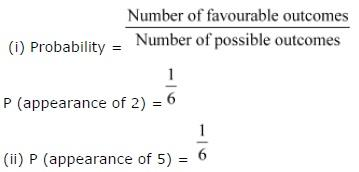Question 3:
A coin is flipped to decide which team starts the game. What is the probability that your team will start?
A coin has two faces − Head and Tail. One team can opt either Head or Tail.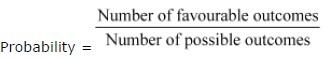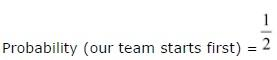Question 4:
A box contains pairs of socks of two colours (black and white). I have picked out a white sock. I pick out one more with my eyes closed. What is the probability that it will make a pair?